## Example Questions

← Previous 1

### Example Question #1 : Negative Numbers

If x is a negative integer, what else must be a negative integer?

x – (–x)

x – x

x² – x

x – (–x)

Explanation:

By choosing a random negative number, for example: –4, we can input the number into each choice and see if we come out with another negative number.  When we put –4 in for x, we would have –4 – (–(–4)) or –4 – 4, which is –8.  Plugging in the other options gives a positive answer.  You can try other negative numbers, if needed, to confirm this still works.

### Example Question #1 : Negative Numbers

–7 – 7= x

–7 – (–7) = y

what are x and y, respectively

x = –14, y = 14

x = –14, y = 0

x = 14, y = –14

y = 0, x = 14

x = 0, y = 0

x = –14, y = 0

Explanation:

x: –7 – 7= –7 + –7 = –14

y: –7 – (–7) = –7 + 7 = 0

when subtracting a negative number, turn it into an addition problem

### Example Question #1 : Negative Numbers

Evaluate: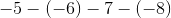Explanation:

Reconvertinto single signs.  Remember that double negatives will turn into a positive.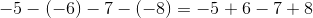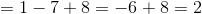### Example Question #2 : Negative Numbers

Subtract: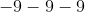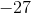Explanation:

Subtracting a negative number moves the number farther left of the zero digit on the number line.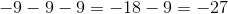The answer is:### Example Question #3 : Negative Numbers

Subtract: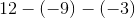Explanation:

Simplify the expression by eliminating the double negative signs.  Two negatives multiplied by one another will result in a positive number.

Convert the signs.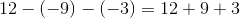Add the right side of the equation.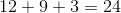The answer is.

### Example Question #4 : Negative Numbers

Simplify the following expression: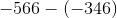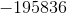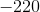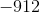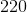Explanation:

Simplify the following expression:To subtract negative numbers, we must recall that subtracting negative numbers is the same as adding a positive. The two negatives cancel each other out to become positive.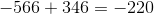So our answer is negative 220

### Example Question #5 : Negative Numbers

If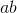is a positive number, and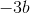is also a positive number, what is a possible value for?Explanation:

Because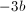is positive,must be negative since the product of two negative numbers is positive.

Because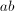is also positive,must also be negative in order to produce a prositive product.

To check you answer, you can try plugging in any negative number for.

### Example Question #11 : Concepts

Multiply the following negative numbers: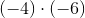.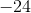Explanation:

When two negative numbers are multiplied by each other, the result is a positive number.

When a negative and a nonnegative number are multiplied with each other, the result is a negative number.

Therefore, sinceandare negative numbers, the product must be positive.

Therefore, the answer is positive.

### Example Question #1 : How To Multiply Negative Numbers

Multiply: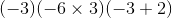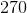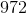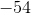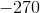Explanation:

Simplify the expression term by term.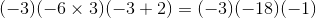A negative number multiplied by a negative number gives a positive number.

A negative number multiplied by a positive number gives a negative number.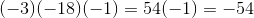### Example Question #2 : How To Multiply Negative Numbers

Multiply: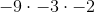Explanation:

Multiply the first two terms.  A negative number times another negative will result in a positive number.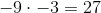Multiply 27 with negative 2.  A positive number times a negative number will result in a negative number.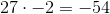The answer is:← Previous 1